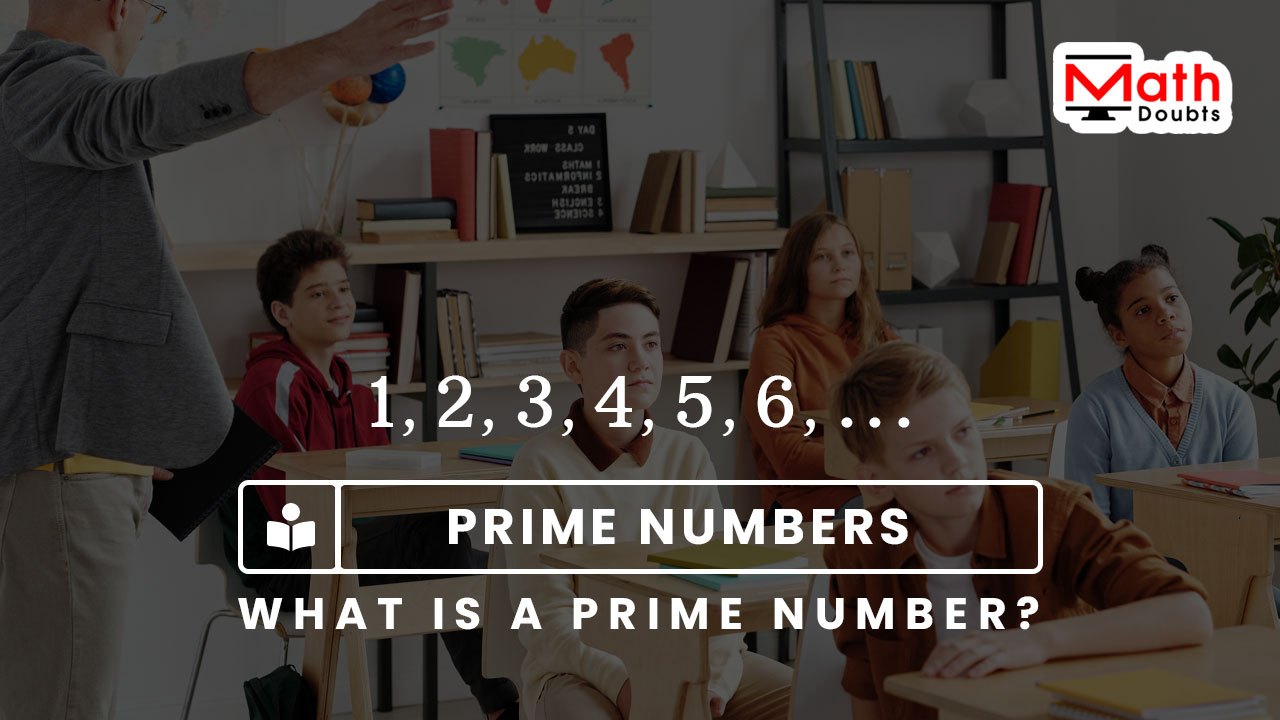# What is a Prime number?

## Definition

A natural number that is divisible only by both one and itself is called a prime number.

### Introduction

There are infinite natural numbers and every natural number is divisible by either one or more natural numbers but some natural numbers, which are primarily divisible only by one and itself. So, those natural numbers are called the prime numbers.Now, let’s learn clearly from some examples that what a prime number is.

$(1).\,\,$ The natural number $1$ is not a prime number.

$\implies$ $1 \div 1$ $\,=\,$ $1$

$(2).\,\,$ The natural number $2$ is a prime number because it is completely divisible only by $1$ and $2$.

$\implies$ $2 \div 1$ $\,=\,$ $2$ and $2 \div 2$ $\,=\,$ $1$

$(3).\,\,$ The natural number $3$ is a prime number because it is completely divisible only by $1$ and $3$.

$\implies$ $3 \div 1$ $\,=\,$ $3$ and $3 \div 3$ $\,=\,$ $1$

$(4).\,\,$ The natural number $4$ is not a prime number because it is completely divisible only by $1$ and $4$ but it is also divisible by $2$.

$\implies$ $4 \div 1$ $\,=\,$ $4,$ $4 \div 2$ $\,=\,$ $2$ and $4 \div 4$ $\,=\,$ $1$

$(5).\,\,$ The natural number $5$ is a prime number because it is completely divisible only by $1$ and $5$.

$\implies$ $5 \div 1$ $\,=\,$ $5$ and $5 \div 5$ $\,=\,$ $1$

The above examples help you to understand the fundamental definition of a prime number. In this way, the prime numbers can be identified from the infinite natural numbers in mathematics.

###### Recommended
Latest Math Topics
Jun 26, 2023
Jun 23, 2023

Latest Math Problems
Jul 01, 2023
Jun 25, 2023
###### Math Questions

The math problems with solutions to learn how to solve a problem.

Learn solutions

Practice now

###### Math Videos

The math videos tutorials with visual graphics to learn every concept.

Watch now

###### Subscribe us

Get the latest math updates from the Math Doubts by subscribing us.